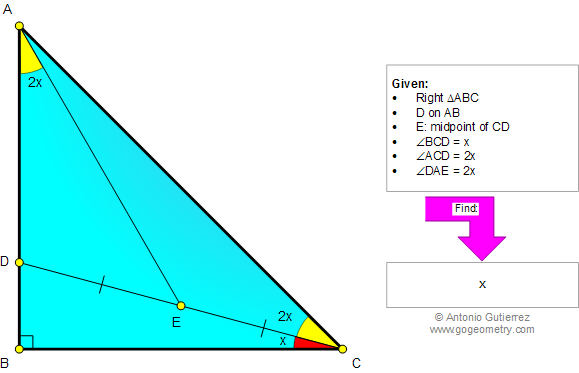# Geometry Problem 999. Right Triangle, Midpoint, Median, Double Angle, Congruence. Level: School, College.

< PREVIOUS PROBLEM  |  NEXT PROBLEM >

 The figure below shows a right triangle ABC, with D on AB and E midpoint of CD. If angle ACD = angle DAE = 2 angle BCD, find the measure of angle BCD. Only elementary geometry is allowed (no trigonometry).Home | SearchGeometry | Problems | All Problems | Open Problems | Visual Index | 10 Problems | Problems Art Gallery Art | 991-1000 | Triangles | Right Triangle | Midpoint | Median | Angles | Double Angle | Congruence | by Antonio Gutierrez